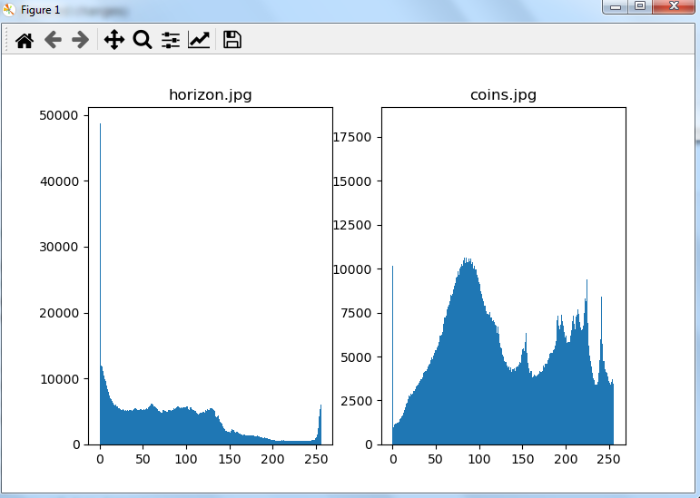# How to compare histograms of two images using OpenCV Python?

The histograms of two images can be compared using cv2.compareHist() function. The cv2.compareHist() function accepts three input arguments- hist1, hist2, and compare_method. The hist1 and hist2 are histograms of the two input images and compare_method is a metric to compute the matching between the histograms. It returns a numerical parameter that expresses how well two histograms match with each other. There are four metrics available to compare the histograms- Correlation, Chi-square, Intersection and Bhattacharyya distance.

## Steps

To compare the histograms of two images one could follow the steps given below −

• Import the required libraries. In all the following Python examples, the required Python libraries are OpenCV and Matplotlib. Make sure you have already installed them.

• Read the input images using cv2.imread() function. Pass the full path of the input image.

• Calculate the histograms of the two input images using cv2.calcHist().

• Normalize the histograms computed above for the two input images using cv2.normalize().

• Compare these normalized histograms using cv2.compareHist(). It returns the comparison metric value. Pass suitable histogram comparison method as parameter to this method.

• Print the histogram comparison metric value. Optionally plot the two histograms for visual understanding.

Let's look at some examples for a clear understanding about the question.

## Input Images

We will use the following images as the input files in the examples below.## Example

In this example, we compare the histograms of two input images. We compute and normalize the histograms of the two images, then compare these normalized histograms.

# import required libraries
import cv2
import matplotlib.pyplot as plt

# Calculate the histograms, and normalize them
hist_img1 = cv2.calcHist([img1], [0, 1, 2], None, [256, 256, 256], [0, 256, 0, 256, 0, 256])
cv2.normalize(hist_img1, hist_img1, alpha=0, beta=1, norm_type=cv2.NORM_MINMAX)
hist_img2 = cv2.calcHist([img2], [0, 1, 2], None, [256, 256, 256], [0, 256, 0, 256, 0, 256])
cv2.normalize(hist_img2, hist_img2, alpha=0, beta=1, norm_type=cv2.NORM_MINMAX)

# Find the metric value
metric_val = cv2.compareHist(hist_img1, hist_img2, cv2.HISTCMP_CORREL)
print("Metric Value:", metric_val)

# plot the histograms of two images
plt.subplot(121), plt.hist(img1.ravel(),256,[0,256]),
plt.title('horizon.jpg')
plt.subplot(122), plt.hist(img2.ravel(),256,[0,256]),
plt.title('coins.jpg')
plt.show()


## Output

On execution, it will produce the following output

Metric Value: -0.13959840937070855


And we get the below window showing the output −## Example

In this example, we compare the histograms of two input images using four different comparison methods: HISTCMP_CORREL, HISTCMP_CHISQR, HISTCMP_INTERSECT, and HISTCMP_BHATTACHARYYA. We compute and normalize the histograms of the two images, then compare these normalized histograms.

# import required libraries
import cv2
import matplotlib.pyplot as plt

# Calculate the histograms, and normalize them
hist_img1 = cv2.calcHist([img1], [0, 1, 2], None, [256, 256, 256], [0, 256, 0, 256, 0, 256])
cv2.normalize(hist_img1, hist_img1, alpha=0, beta=1, norm_type=cv2.NORM_MINMAX)
hist_img2 = cv2.calcHist([img2], [0, 1, 2], None, [256, 256, 256], [0, 256, 0, 256, 0, 256])
cv2.normalize(hist_img2, hist_img2, alpha=0, beta=1, norm_type=cv2.NORM_MINMAX)

# Find the metric value
metric_val1 = cv2.compareHist(hist_img1, hist_img2, cv2.HISTCMP_CORREL)
metric_val2 = cv2.compareHist(hist_img1, hist_img2, cv2.HISTCMP_CHISQR)
metric_val3 = cv2.compareHist(hist_img1, hist_img2, cv2.HISTCMP_INTERSECT)
metric_val4 = cv2.compareHist(hist_img1, hist_img2, cv2.HISTCMP_BHATTACHARYYA)
print("Metric Value using Correlation Hist Comp Method", metric_val1)
print("Metric Value using Chi Square Hist Comp Method", metric_val2)
print("Metric Value using Intersection Hist Comp Method", metric_val3)
print("Metric Value using Bhattacharyya Hist Comp Method", metric_val4)


## Output

On execution, it will produce the following output

 Metric Value using Correlation Hist Comp Method: -0.13959840937070855
Metric Value using Chi Square Hist Comp Method: 763.9389629197002
Metric Value using Intersection Hist Comp Method: 6.9374825183767825
Metric Value using Bhattacharyya Hist Comp Method: 0.9767985879980464


Updated on: 02-Dec-2022

3K+ Views